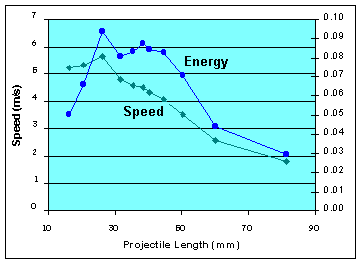# Mark II Results - Projectile Length

The projectile length affects performance, but how? Shorter projectiles have greater acceleration for the same force, but they also have less material to interact with the magnetic field. And if they do go faster, do they carry more or less kinetic energy than longer projectiles?

## Length of Projectile

I measured the performance of projectiles of eleven different lengths, ranging from 15mm to 80mm. I calculated the average muzzle velocity (m/s) and kinetic energy (Joules) for each projectile, shown on the graph at right.Length Speed Energy
16.3mm  5.24 ms/s  0.0501 J
20.6 5.34 0.0659
26.2 5.64 0.0936
31.5 4.78 0.0808
35.4 4.58 0.0832
38.4 4.51 0.0875
40.3 4.32 0.0845
44.7 4.07 0.0831
50.3 3.53 0.0704
60.0 2.56 0.0442
81.3 1.80 0.0295

It is important to adjust the starting position for each projectile. As discovered in previous tests, the exit speed is rather sensitive to the particular starting position. There is a range of 6mm which permits operation, and an ideal range of only 2mm of variation in initial placement. Since the length difference between projectiles was generally 5mm, some adjustment is needed for each one. I arranged it so the front of each projectile was inserted the same (6mm) amount into the coil. This gave quite satisfactory results, saving the effort of re-discovering the optimum starting position for each test.

My testing made at least five measurements from each projectile and averaged the results. Note there was often a 10% speed variation between shots using an identical setup. It would be instructive to analyze the variance, but that was more work than I cared for.

## Conclusions

Shorter projectiles went faster than longer ones. The fastest projectile was 26mm long, and it flew at 5.6 m/s when driven at 23 volts.

There is what might be a linear relation between speed and projectile length, of roughly v = (-0.0688)x + 7.096, where v is speed in m/s and x is length in mm. This seems to hold for projectiles in the range -25% to +50% of the coil length. The data could also match an inverse relationship (which would be consistent with a constant energy transfer), but the data is not smooth enough to be conclusive.

The energy curve is interesting. The kinetic energy stored in the projectile forms a hump which is within 10% of flat for projectiles in the range -25% to +25% of the coil length. There seems to be a constant energy transfer for projectiles nearly the same length as the coil. If not exactly constant, it is enough to conclude coilguns are not sensitive to projectile length over this small range.

 < Previous Page 15 of 18 Next >